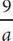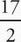# SAT Math Multiple Choice Question 193: Answer and Explanation

### Test Information

Question: 193

13. V(t) = at + k

At a certain manufacturing plant, the total number of vacation days, V(t), an employee has accrued is given by the function above, where t is the number of years the employee has worked at the plant, and a and k are constants. If Martin has accrued 9 more vacation days than Emilio has, how many more years has Martin worked than Emilio?

• A.• B. 9 - a
• C. 9 + a
• D. 9a

A Whenever there are variables in the question and in the answers, think Plugging In. Let's say that for Emilio a = 2, t = 4, and k = 10. Then Emilio's accrued vacation days can be calculated as V(t) = 2(4) + 10 = 18. This means that Martin has accrued 18 + 9 = 27 vacation days. Because a and k are constants, their values do not change. The number of years that Martin has worked at the manufacturing plant can therefore be calculated as 27 = 2t + 10. Solve for t to get 17 = 2t or t == 8.5. Therefore, Martin has worked 8.5 - 4 = 4.5 more years than Emilio. Plug 2 in for a in the answers to see which answer equals 4.5. Choice (A) becomes= 4.5. Keep (A) but check the remaining answers just in case. Choice (B) becomes 9 - 2 = 7, (C) becomes 9 + 2 = 11, and (D) becomes 9(2) = 19. Eliminate (B), (C), and (D). The correct answer is (A).